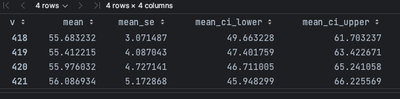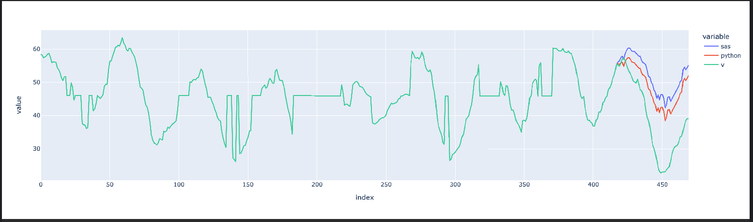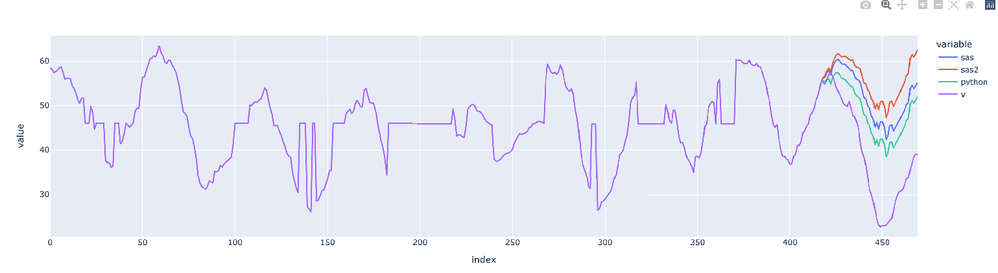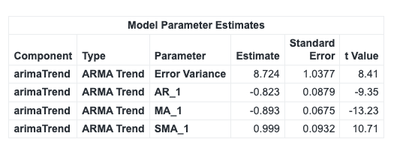## uniTimeSeries.arima results are different (and worse) than statsmodels SARIMA

Hi, I have a very simple time series (attached) which I need to model and forecast with sarima. The model is already defined: p,d,q=(1,1,1), the seasonality is 52, and the p,d,q for seasonality are = (0,1,1).

I fit the model with python statsmodels library SARIMAX:

```import pandas as pd
from statsmodels.tsa.statespace.sarimax import SARIMAX
model_training = SARIMAX(df.v, order=(1, 1, 1), seasonal_order=(0, 1, 1, 52)).fit(disp=False)
model_training.get_forecast(1).summary_frame()
```

and I get for example the first forecast value:

```mean	        mean_se	        mean_ci_lower	mean_ci_upper
55.683232	3.071487	49.663228	61.703237
```

Then I fit the very same model with the very same data in CAS using uniTimeSeries.arima:

``````data series;
infile "series.csv" dsd truncover firstobs=2 delimiter=";";
input d \$ v;
date = input(d,ddmmyy10.);
format date yymmdd10.;
drop d;
rename date=d;
run;

cas session;
libname CASUSER cas caslib="CASUSER";
data CASUSER.series; set series; run;

proc cas;
uniTimeSeries.arima /
table={name="series", caslib="CASUSER"}
timeId={name="d"}
interval="day"
outFor={name="for", replace=True}
outEst={name="est", replace=True}
series={{name='v',
model={{estimate={p={{factor={1}}} q={{factor={1,52}}},diff={1, 52},noint=True},
}}
;
run;
quit;``````

and I get for example the first forecast value:

```Forecast	Std Error	95% Confidence Limits
56.4786	        3.5467	        49.5272	63.4301```

As you can see the forecasted value is different, and the SAS model is generally worse: the true value is closer to 55, and also the CL are broader. And it gets worse requesting more forecast leads, while the python model keeps being more accurate.

My question is: why? How it is possible that the SAS model has a different result? I tried to change maybe the noint parameter or the convergence criteria to see maybe if there were some different defaults, but no matter what I change the python model is always better.

What am I missing?

Just to contextualize: in the project I'm following, the team has already experimented successfully with python statsmodels for some time series; now they want to apply the model to thousands of time series exploiting the CAS parallelism, but they are being very disappointed since the models they already know were good are not goot anymore in sas, and I really don't understand why.

Note: my final model must run in CAS, so please do not provide sas base code.

Thanks a lot!

1 ACCEPTED SOLUTION

Accepted Solutions

## Re: uniTimeSeries.arima results are different (and worse) than statsmodels SARIMA

``````data series;
infile "sasuser/series.csv" dsd truncover firstobs=2 delimiter=";";
input d \$ v;
date = input(d,ddmmyy10.);
format date yymmdd10.;
drop d;
rename date=d;
run;
/* NOTE that you can use observation numbers */
data series;
set work.series;
index = d - '01JAN2022'D;
run;
dataseries_ext;
do index = 418 to 469;
v=.;
output;
end;
run;
data series_ext;
set series series_ext;
run;

title "ssm";
proc ssm data=series_ext;
id index interval=obs;
*trend arimaTrend(arma(d=1 sd=1 p=1 q=1 sq=1 s=52)) ;
trend arimaTrend(arma(d=1 sd=1 p=1 q=1 sq=1 s=52)) ar=0.8550 ma=-0.9781 -0.9990  ;
model v = arimaTrend ;
output outfor=For;
run;

proc print data=For;
run;
``````

Left out the code to extend the series with missing in order to obtain forecast values.

15 REPLIES 15

## Re: uniTimeSeries.arima results are different (and worse) than statsmodels SARIMA

Hello,

And for the model specification, I think you need to do it this way :

``````series={{name='v',
model={{ estimate={p={{factor={1}}}
q={{factor={1}}, {factor={52}}},
method='ML',
diff={1, 52},
noint=True},
}}``````

[EDIT] :
PROC ARIMA will run in SPRE indeed
, but you can also run PROC CARIMA (SAS Econometrics).
PROC CARIMA will run in CAS as it is a so-called CAS-enabled procedure (no need to use PROC CAS).
https://go.documentation.sas.com/doc/en/pgmsascdc/v_031/casecon/casecon_carima_toc.htm

[EXTRA EDIT]

What is a CAS-enabled procedure?
By Rick Wicklin on The DO Loop November 22, 2021
https://blogs.sas.com/content/iml/2021/11/22/cas-enabled-procedure.html

BR,

Koen

## Re: uniTimeSeries.arima results are different (and worse) than statsmodels SARIMA

Thanks, with your model specification it is getting a little bit closer, but still is much worse than python, I really can't figure out why:

SAS:PYTHONHere's a comparison on 52 steps forecast:As you can see python and sas predictions seem to have a similar shape but sas is off of some kind of weird offset (around -3, but variable it is not a constant).

And also the sas 95% confidence interval is way broader than python one (at step 52, [11.6813, 98.6936] vs [37.640213, 66.402516])

About the interval it doesn't really matter, yes the data are weekly (hence the '52') but they already are processed (averaged, missing filled, etc) so it is just a sequence of numbers, dates don't matter nor the interval. In fact, in python I even didn't use the fake date column, while sas needs the interval= parameter so I just provided a fake incremental date with interval=day.

What do you think?

Thanks a lot again,

Regards

## Re: uniTimeSeries.arima results are different (and worse) than statsmodels SARIMA

Hello,

You can only use the uniTimeSeries.arima action if you have SAS Econometrics (SAS VIYA).

So you also have PROC CARIMA (which is 100% CAS-enabled).
PROC CARIMA is probably calling the uniTimeSeries.arima action in the background.

But I know PROC CARIMA syntax better. Hence, I shift to PROC CARIMA. 😊

Can you try below code?

``````libname mycas cas caslib=casuser;

data mycas.series(replace=YES); set work.series; run;

proc carima data=mycas.series outest=mycas.cariest outfor=mycas.carifor;
id date interval=day; /* your date/index variable should be like row numbers (interval=1) */
identify v;
estimate p=(1) q=(1)(52) NOINT=0 method = ML
/* transform = log */ diff = (1,52)
CONVERGE=0.0005 MAXITER=500 NOSTABLE=1;
run;
/* end of program */``````

Here's some DOC on PROC CARIMA.
https://go.documentation.sas.com/doc/en/pgmsascdc/v_031/casecon/casecon_carima_toc.htm

Thanks,

Koen

## Re: uniTimeSeries.arima results are different (and worse) than statsmodels SARIMA

Thank you for your answer, yes afaik proc carima and uniTimeSeries.arima are the same, I've been using the latter because at the end I will call it with API, but proc carima code is totally ok (I will translate it later).

So I tried your new model specification, and it gets even worse ("sas2" is this new model):Changing some parameters just to experiment with, I see that "maxiter" and "converge" have no effect on the result, while "noint" if is false produces the "sas2" forecast, while if is true produces the "sas" forecast, so without the intercept seems closer to python (I saw that python SARIMAX doesn't use the intercept by default).

It seems so weird, that particular shape you can see at 450 is present both in python and sas, it seems they are doing the same calculation, but sas one has this mysterious "trend" or "offset" upward...

## Re: uniTimeSeries.arima results are different (and worse) than statsmodels SARIMA

Hello,

... or Python has this mysterious "trend" or "offset" downward (which happens to be in your favour this time)?

I thought the difference was in the NOSTABLE option, but apparently not.

NOSTABLE=0 | 1

... specifies whether to restrict the candidate estimates for the autoregressive and moving average parameters in the stationary and invertible regions, respectively. You can specify the following values:
0 : restrict candidate estimates in stable and invertible regions.
1 : do not restrict candidate estimates.
By default, NOSTABLE=0. (I would always leave it on "0" by the way, the "1" was just an attempt to mimic Python)

What if you compare the parameter estimates instead of comparing the forecasts?
Are the estimates close to each other (Python versus SAS)?

ARIMA is dealt with in SAS since the mid 80's or so. And it has been used A LOT (!! A LOT !!) since then.
I consider it close to impossible that there would be a flaw in its implementation. And certainly not with such a simple model specification.
Maybe Python has some hidden transformations that it tries out (and it then reverts back to the original scale)??

I do not know how to proceed ☹️.

Maybe @SASCom1 can help you out , but I am afraid she will only return to the office on January 10th (a Tuesday).
I will contact another colleague internally to see if she can help.

Good luck,
Koen

## Re: uniTimeSeries.arima results are different (and worse) than statsmodels SARIMA

Yes of course it may be some mysterious trick from statsmodels implementation 😁 I tried to read their documentation as well and dig in their code being open source, so far I didn't figure out anything special.

Naturally I don't doubt neither sas nor python implementations, I believe they are very robust, I just need to find the reason behind the models difference to be able to explain it to my business.

When I ported their models to sas I didn't even check the results since I believed they would have been equals by default, but then they pointed out that there are several series where the difference is quite strong (measured i.e. with rmse, python ones are smaller), so I took this series as an example and started to dig deeper isolating the code to try to figure out why.

Trying to compare the coefficients as you suggest, I tried to insert one pdq at a time in both models and comparing coefficient estimates each time. I found out that up to the simple model (1,0,1) they are approximately the same, when adding (1,1,1) they begin to be different and also when adding seasonality (1,0,1)x(0,0,1)52 they are different; mixing differenciating with seasonality to build the final model (1,1,1)x(0,1,1)52 they are a lot different.

Another test I tried: rewriting the model with proc tsmodel; the results are identical to proc carima/unitimeseries.arima.

Thanks a lot for your time and your help, very much appreciated.

Regards

## Re: uniTimeSeries.arima results are different (and worse) than statsmodels SARIMA

Hello,

You used statsmodels Python library.
Can you try another Python library??

Here are 𝟏𝟎 𝐓𝐢𝐦𝐞-𝐒𝐞𝐫𝐢𝐞𝐬 𝐏𝐲𝐭𝐡𝐨𝐧 𝐥𝐢𝐛𝐫𝐚𝐫𝐢𝐞𝐬 𝐢𝐧 𝟐𝟎𝟐𝟐 .
The first 3 (in green) can do (S)ARIMA(X) for sure ! For the others, I don't know.

• Darts
• Pmdarima
• Statsforecast
• Flow forecast
• Auto_TS
• SKTIME
• TSFresh
• Pyflux
• Prophet
• PyCaret
• ( NeuralProphet )

Cheers,
Koen

## Re: uniTimeSeries.arima results are different (and worse) than statsmodels SARIMA

Since SARIMAX uses a statespace representation, you might find it easier to compare using the ssm procedure. For example, this code gives very similar forecasts.

``````data series;
infile "sasuser/series.csv" dsd truncover firstobs=2 delimiter=";";
input d \$ v;
date = input(d,ddmmyy10.);
format date yymmdd10.;
drop d;
rename date=d;
run;
/* NOTE that you can use observation numbers */
data series;
set work.series;
index = d - '01JAN2022'D;
run;
title "ssm";
proc ssm data=series_ext;
id index interval=obs;
*trend arimaTrend(arma(d=1 sd=1 p=1 q=1 sq=1 s=52)) ;
trend arimaTrend(arma(d=1 sd=1 p=1 q=1 sq=1 s=52)) ar=0.8550 ma=-0.9781 -0.9990  ;
model v = arimaTrend ;
output outfor=For;
run;

proc print data=For;
run;

``````

## Re: uniTimeSeries.arima results are different (and worse) than statsmodels SARIMA

``````data series;
infile "sasuser/series.csv" dsd truncover firstobs=2 delimiter=";";
input d \$ v;
date = input(d,ddmmyy10.);
format date yymmdd10.;
drop d;
rename date=d;
run;
/* NOTE that you can use observation numbers */
data series;
set work.series;
index = d - '01JAN2022'D;
run;
dataseries_ext;
do index = 418 to 469;
v=.;
output;
end;
run;
data series_ext;
set series series_ext;
run;

title "ssm";
proc ssm data=series_ext;
id index interval=obs;
*trend arimaTrend(arma(d=1 sd=1 p=1 q=1 sq=1 s=52)) ;
trend arimaTrend(arma(d=1 sd=1 p=1 q=1 sq=1 s=52)) ar=0.8550 ma=-0.9781 -0.9990  ;
model v = arimaTrend ;
output outfor=For;
run;

proc print data=For;
run;
``````

Left out the code to extend the series with missing in order to obtain forecast values.

## Re: uniTimeSeries.arima results are different (and worse) than statsmodels SARIMA

Thank you VERY much for your answer, that seems the key to understand the difference between the two models.

Just one question: the coefficients you got

``ar=0.8550 ma=-0.9781 -0.9990``

are indeed very close to the coefficients I get with statsmodels (and other libraries, i.e. the above mentioned darts); however how did you find them out?

Just running:

``trend arimaTrend(arma(d=1 sd=1 p=1 q=1 sq=1 s=52)) ;``which are very close to the original proc carima results (in fact I get the same prediction as proc carima, not the state space one).

What am I missing?

Thanks a lot again,

Regards

## Re: uniTimeSeries.arima results are different (and worse) than statsmodels SARIMA

The coefficients are taken from the python SARIMA output, and used as input to SAS SSM, then the forecasts are verified. However, from this point, you might be able to adjust the other options until you get the same model identified/estimated.

## Re: uniTimeSeries.arima results are different (and worse) than statsmodels SARIMA

Thanks, I'm trying changing for example the optimizer and the maximum iterations, sometimes I get a little bit closer sometimes not, so far I'm not succeeding in finding the corresponding config but I'll keep trying 🙂

## Re: uniTimeSeries.arima results are different (and worse) than statsmodels SARIMA

I am adding some code to this discussion that should clarify things a little.  I am going to use PROC ARIMA rather than CARIMA because you can fix parameter values in ARIMA but CARIMA doc does not show any way to do that (otherwise, the results should match because they both use the same underlying code).

1. PROC ARIMA code for the model ARIMA (1,1,1)(0,1,1)52 NOINT model:

proc arima data=test;
identify var=v(1, 52) noprint;
estimate p=1 q=(1)(52) noint method=ml;

quit;

For these data the parameter estimation leads to an unstable model (the seasonal MA parameter is close to the boundary).  Forecast at the end is 56.2380.  The parameter estimates are different from the SARIMA model estimates (shown in Tammy's code).

2.  PROC ARIMA code for the model ARIMA (1,1,1)(0,1,1)52 NOINT model with parameters fixed at SARIMA estimates (note that the sign convention of SARIMA and PROC ARIMA appears to be opposite for MA parameters):

proc arima data=test;
i var=v(1, 52) noprint;
e p=1 q=(1)(52) noint method=ml ar=0.8550 ma=0.9781 0.9990 noest;
quit;

Now the forecast at the end is 55.6832, which essentially is the same as the SARIMA forecast.  By the way, SARIMA estimates also suggest an unstable model.

So, in fact, both SARIMA and PROC ARIMA are doing the same likelihood and forecast calculations but for many problematic optimization situations the nonlinear optimization processes can lead to different final results.  This is the case in this particular data/model situation.

Hopefully this helps.

Discussion stats
• 15 replies
• 1353 views
• 7 likes
• 4 in conversation